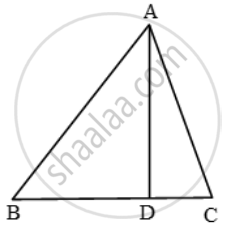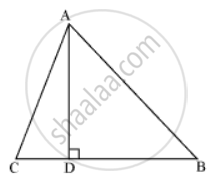# The Perpendicular Ad on the Base Bc of a ∆Abc Intersects Bc at D So that Db = 3 Cd. Prove that 2 Ab 2 = 2 Ac 2 + Bc 2 - Mathematics

Sum

The perpendicular AD on the base BC of a ∆ABC intersects BC at D so that DB = 3 CD. Prove that 2"AB"^2 = 2"AC"^2 + "BC"^2

#### Solution 1

We haveDB = 3CD

BC = BD + DC

The perpendicular AD on the base BC of a ∆ABC intersects BC at D so that DB = 3 CD. Prove that 2AC2 + BC2.

We have,

DB = 3CD

∴ BC = BD + DC

⇒ BC = 3 CD + CD

⇒ BD = 4 CD ⇒ CD = \frac { 1 }{ 4 } BC

∴ CD = \frac { 1 }{ 4 } BC and BD = 3CD = \frac { 1 }{ 4 } BC  ….(i)

Since ∆ABD is a right triangle right-angled at D.

∴ AB^2 = AD^2 + BD^2 ….(ii)

Similarly, ∆ACD is a right triangle right angled at D.

∴ AC^2 = AD^2 + CD^2 ….(iii)

Subtracting equation (iii) from equation (ii) we get

AB^2 – AC^2 = BD^2 – CD^2

⇒ AB^2 – AC^2 = ( \frac{3}{4}BC)^{2}-( \frac{1}{4}BC)^{2}[

⇒ AB^2 – AC^2 = \frac { 9 }{ 16 } BC^2 – \frac { 1 }{ 16 } BC^2

⇒ AB^2 – AC^2 = \frac { 1 }{ 2 } BC^2

⇒ 2(AB^2 – AC^2 ) = BC^2

⇒ 2AB^2 = 2AC^2 + BC^2

#### Solution 2In ΔACD
AD2 = AC2 - DC2     ...(1)
In ΔABD
AD2 = AB2 - DB2     ...(2)
From equation (1) and (2)
Therefore AC2 - DC2 = AB2 - DB2
since given that 3DC = DB

DC = "BC"/(4) and "DB" = (3"BC")/(4)

"AC"^2 - ("BC"/4)^2 = "AB"^2 - ((3"BC")/4)^2

"AC"^2 - "Bc"^2/(16) = "AB"^2 - (9"BC"^2)/(16)

16AC2 - BC2 = 16AB2 - 9BC2
⇒ 16AB2 - 16AC2 = 8BC2
⇒ 2AB2 = 2AC2 + BC2.

Concept: Right-angled Triangles and Pythagoras Property
Is there an error in this question or solution?

#### APPEARS IN

Frank Class 9 Maths ICSE
Chapter 17 Pythagoras Theorem
Exercise 17.1 | Q 19